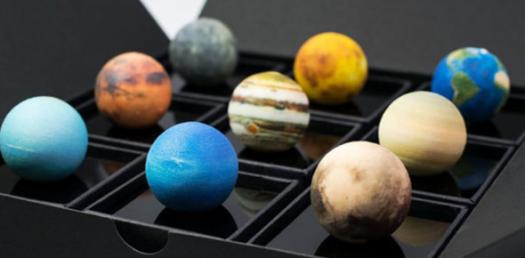# Quiz: Test Your Knowledge On The Solar System!

10 Questions | Total Attempts: 1242Settings• 1.
How many stars are their in our solar system?
• A.

1

• B.

2

• C.

3

• D.

Billions

• 2.
The current model of the solar system is called the heliocentric model of the solar system.
• A.

True

• B.

False

• 3.
How long does it take the earth to go around the sun one time?
• A.

24 days

• B.

24 hours

• C.

365 days

• D.

365 hours

• 4.
How long does it take the earth to spin around one time?
• A.

24 days

• B.

24 hours

• C.

365 days

• D.

365 hours

• 5.
The two forces that keep the earth in orbit of the sun are?
• A.

Rotation and revolution

• B.

Mass and acceleration

• C.

Inertia and gravity

• D.

Gravity and air resistance

• 6.
How long does it take the moon to revolve around the earth?
• A.

1 day

• B.

1 month

• C.

1 year

• D.

365 days

• 7.
A lunar eclipse can only be seen during a new moon phase?
• A.

True

• B.

False

• 8.
The term for the moon getting brighter (more light) each day is?
• A.

Waxing

• B.

Waning

• C.

Gravity

• D.

Inertia

• 9.
Pluto is a planet.
• A.

True

• B.

False

• 10.
The best science teacher in the world is Mr. D'Alsace.
• A.

True

• B.

False

Related TopicsBack to top